# Steve Butler - UCLA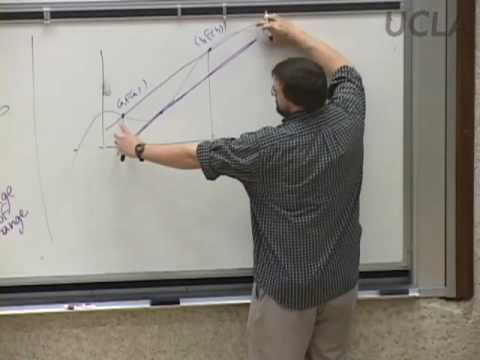#### Differential & Integral Calculus, Lec 12, Math 31A

Topic: Calculus
Teacher: Steve Butler
Concepts In This Lecture: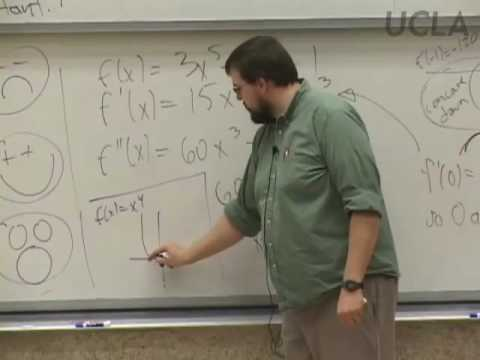#### Differential & Integral Calculus, Lec 13, Math 31A

Topic: Calculus
Teacher: Steve Butler
Concepts In This Lecture: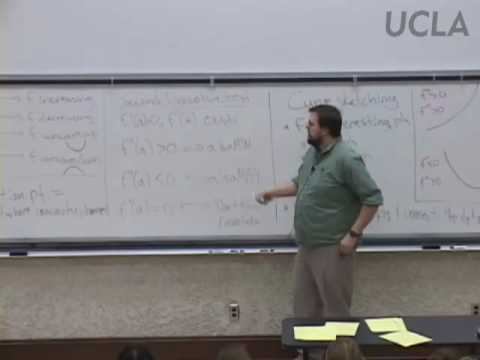#### Differential & Integral Calculus, Lec 14, Math 31A

Topic: Calculus
Teacher: Steve Butler
Concepts In This Lecture: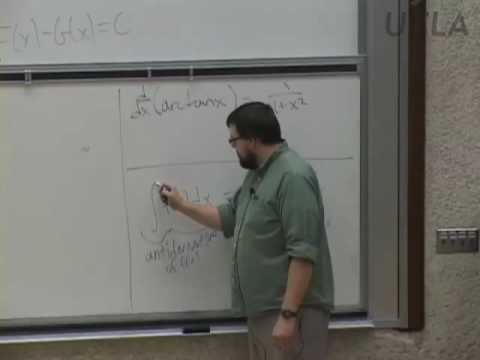#### Differential & Integral Calculus, Lec 15, Math 31A

Topic: Calculus
Teacher: Steve Butler
Concepts In This Lecture: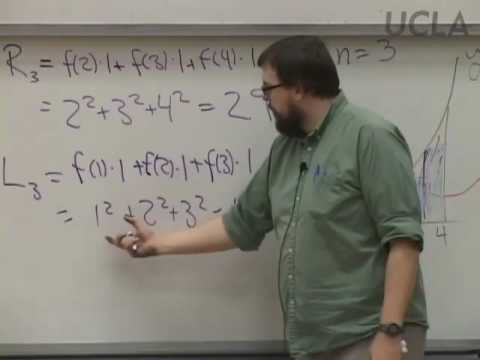#### Differential & Integral Calculus, Lec 16, Math 31A

Topic: Calculus
Teacher: Steve Butler
Concepts In This Lecture: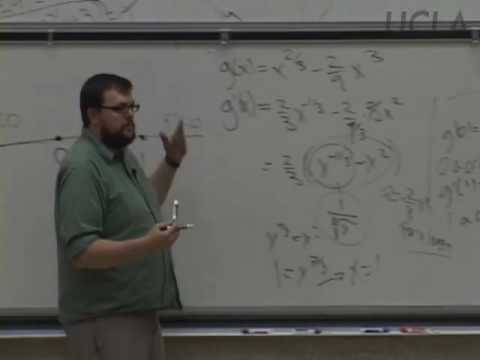#### Differential & Integral Calculus, Lec 11, Math 31A

Topic: Calculus
Teacher: Steve Butler
Concepts In This Lecture: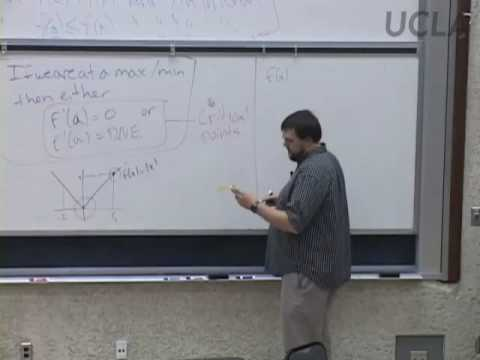#### Differential & Integral Calculus, Lec 10, Math 31A

Topic: Calculus
Teacher: Steve Butler
Concepts In This Lecture: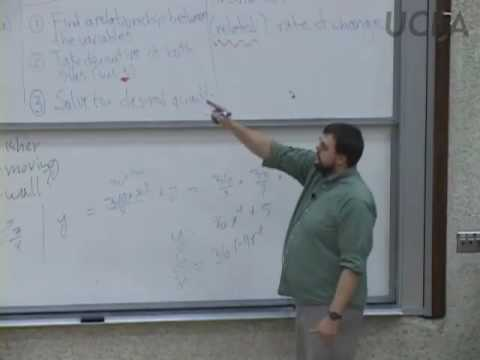#### Differential & Integral Calculus, Lec 9, Math 31A

Topic: Calculus
Teacher: Steve Butler
Concepts In This Lecture: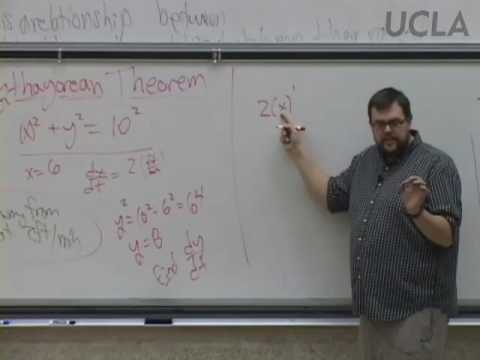#### Differential & Integral Calculus, Lec 8, Math 31A

Topic: Calculus
Teacher: Steve Butler
Concepts In This Lecture: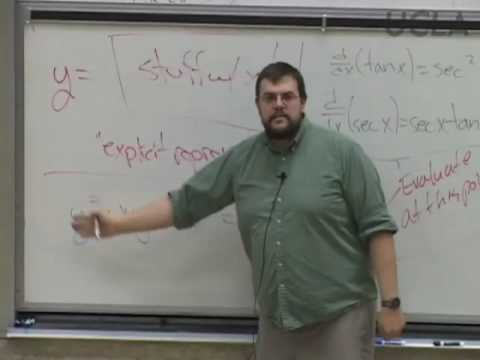#### Differential & Integral Calculus, Lec 7, Math 31A

Topic: Calculus
Teacher: Steve Butler
Concepts In This Lecture: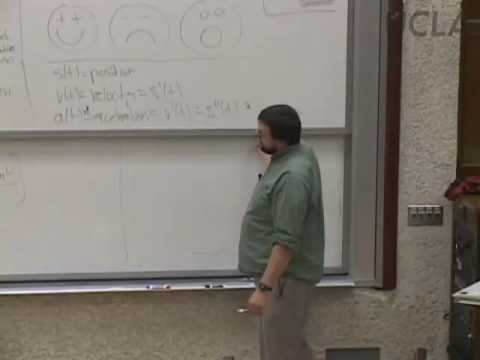#### Differential & Integral Calculus, Lec 6, Math 31A

Topic: Calculus
Teacher: Steve Butler
Concepts In This Lecture: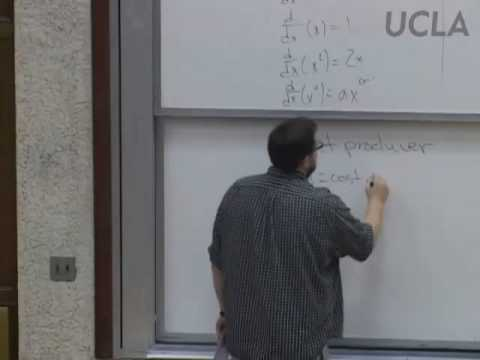#### Differential & Integral Calculus, Lec 5, Math 31A

Topic: Calculus
Teacher: Steve Butler
Concepts In This Lecture: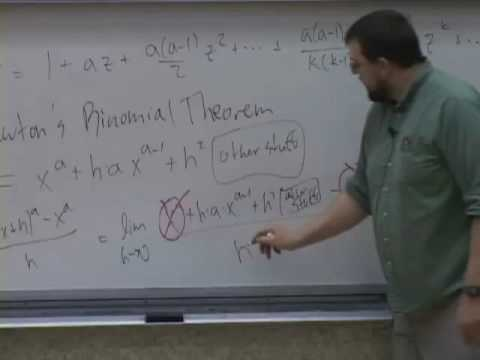#### Differential & Integral Calculus, Lec 4, Math 31A

Topic: Calculus
Teacher: Steve Butler
Concepts In This Lecture: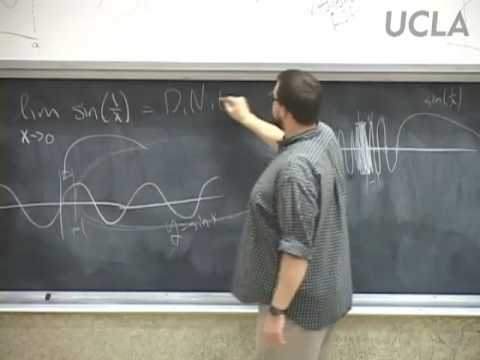#### Differential & Integral Calculus, Lec 2, Math 31A

Topic: Calculus
Teacher: Steve Butler
Concepts In This Lecture: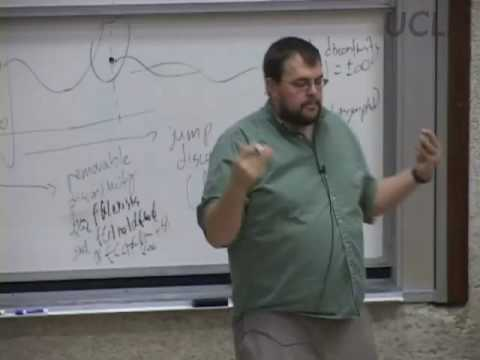#### Differential & Integral Calculus, Lec 3, Math 31A

Topic: Calculus
Teacher: Steve Butler
Concepts In This Lecture: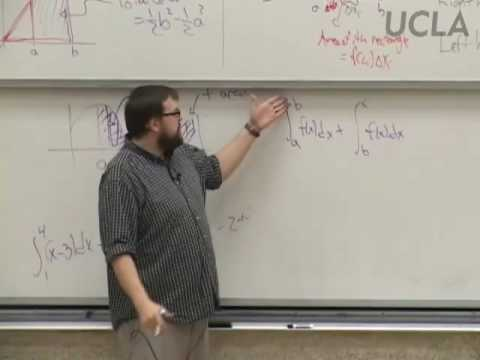#### Differential & Integral Calculus, Lec 17, Math 31A

Topic: Calculus
Teacher: Steve Butler
Concepts In This Lecture: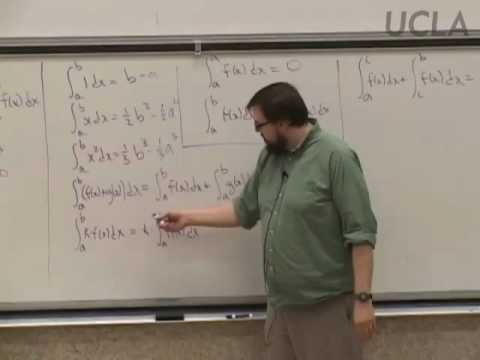#### Differential & Integral Calculus, Lec 18, Math 31A

Topic: Calculus
Teacher: Steve Butler
Concepts In This Lecture: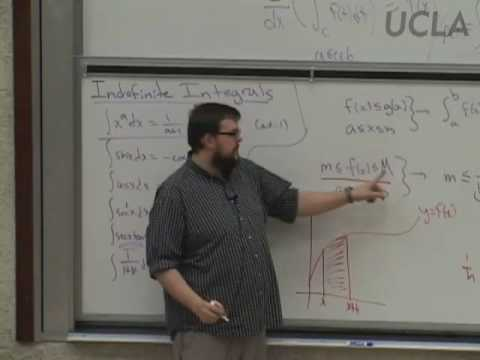#### Differential & Integral Calculus, Lec 19, Math 31A

Topic: Calculus
Teacher: Steve Butler
Concepts In This Lecture: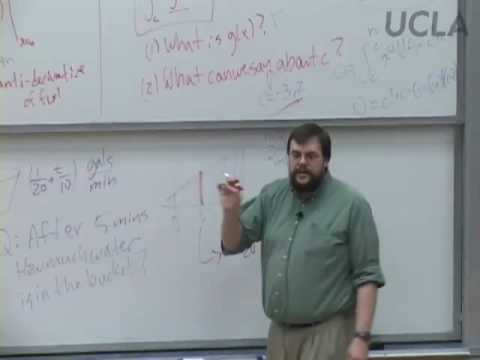#### Differential & Integral Calculus, Lec 20, Math 31A

Topic: Calculus
Teacher: Steve Butler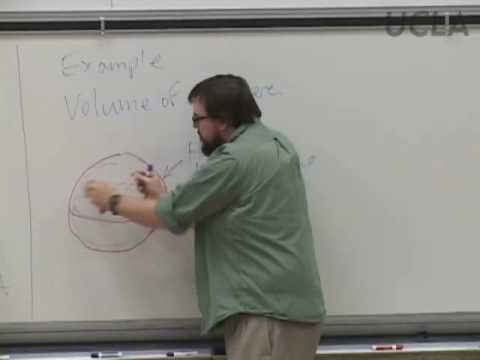#### Differential & Integral Calculus, Lec 21, Math 31A

Topic: Calculus
Teacher: Steve Butler
Concepts In This Lecture: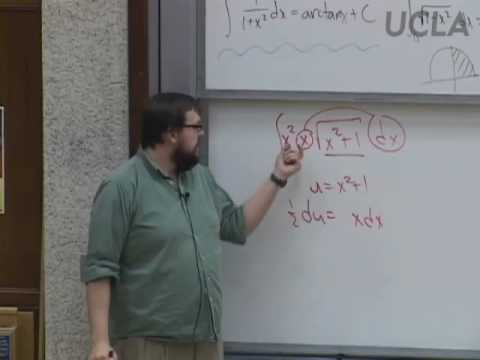#### Differential & Integral Calculus, Lec 22, Math 31A

Topic: Calculus
Teacher: Steve Butler
Concepts In This Lecture: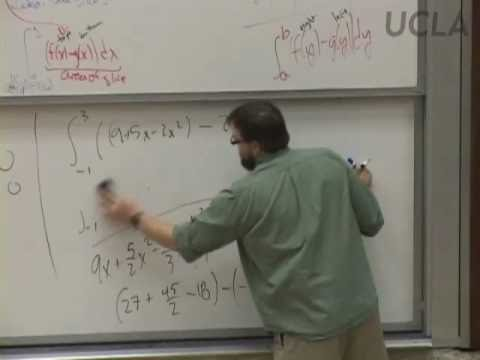#### Differential & Integral Calculus, Lec 23, Math 31A

Topic: Calculus
Teacher: Steve Butler
Concepts In This Lecture: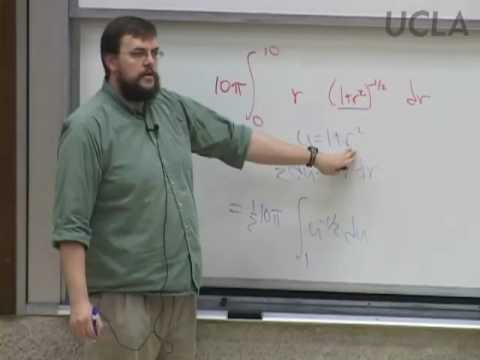#### Differential & Integral Calculus, Lec 24, Math 31A

Topic: Calculus
Teacher: Steve Butler
Concepts In This Lecture: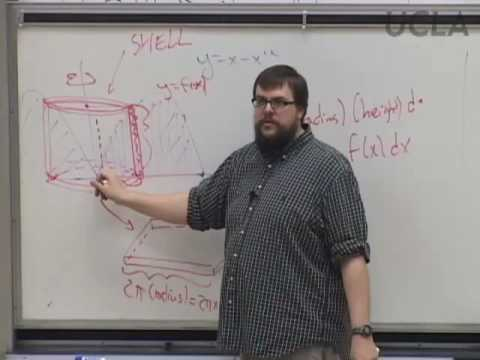#### Differential & Integral Calculus, Lec 25, Math 31A

Topic: Calculus
Teacher: Steve Butler
Concepts In This Lecture: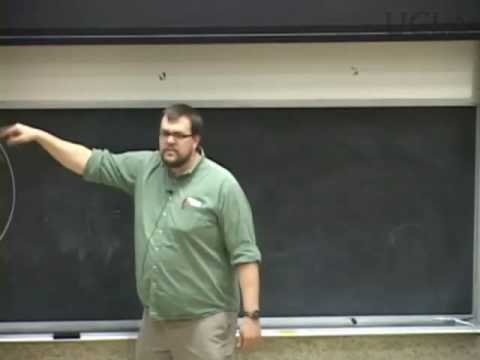#### Differential & Integral Calculus, Lec 1, Math 31A

Topic: Calculus
Teacher: Steve Butler

#### Our Philosophy

TeachingTree is an open platform that lets anybody organize educational content. Our goal is for students to quickly access the exact clips they need in order to learn individual concepts. Everyone is encouraged to help by adding videos or tagging concepts. Read our about page for more info.

#### Talk To Us

If you have any suggestions for how to make the TeachingTree better, questions about how to use the site, or just want to chat, email whatsup@teachingtree.co and we'll get back to you. We're always happy to talk.